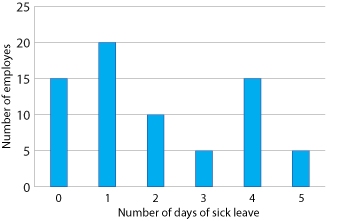## Median

Another measure of central tendency of a data set is the median. One common use is to describe the housing market in a city. The median is the middle value when all the values are arranged in numerical order.

#### Example 5

This data set has been arranged in numerical order:

 2 2 3 3 3 4 5 11 13 18 18 19 21 Median

This data set has an odd number of values. The middle value is 5 since it has the same number of values on either side of it. Hence the median of the data set is 5.

#### Example 6

Here is another data set arranged in numerical order.

 1 3 4 4 5 7 9 11 13 13 19 21 Median

This data set has an even number of values, the middle two of which are 7 and 9. The median is the average (or mean) of these two numbers.

\begin{align}\text{Median}\ &= \dfrac{7 + 9} {2}\\\\ &= 8\end{align}

Notice that this value does not appear in the data set.

So, in summary, to calculate the median of a data set:

• when the data set has an odd number of values and they are arranged in numerical order, the median is the middle value
• when the data set has an even number of values and they are arranged in numerical order, the median is the average of the two middle values.

### Median when data is presented in a stem-and-leaf plot

#### Example 7

The stem-and-leaf plot below shows the approximate weights of students in a class. Determine the median weight of the students.

 Frequency 4 4 6 7 7 8 5 5 2 4 5 5 6 8 8 9 8 6 0 2 4 5 7 9 6 7 0 2 6$$\mid$$4 means 64 kg 2 Total 21

#### Solution

The frequency column has been added to the stem-and-leaf plot and we can see that 21 (an odd number) weights have been recorded. So the median will be the weight in position 11 (giving ten weights on either side). This will be the sixth value in the second class. Hence the median weight is 58 kg.

### Median when data is presented in a column graph

#### Example 8

The column graph below gives the number of days sick leave taken by employees at a factory during a particular month. Locate the median.Detailed description

#### Solution

From the column graph, there are 15 + 20 + 10 + 5 + 15 + 5 = 70 data values, so the median will be the average of the 35th and 36th positions.

The 35th position is a 1 (there are 15 zeroes and 20 ones) and the 36th position is a 2, so

\begin{align}\text{Median}\ &=\ \dfrac{1 + 2}{2}\\\\ &=\ 1.5\ \text{days of sick leave}\end{align}